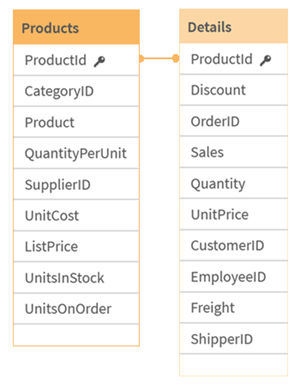Aggregation functions

# Aggregation functions

The family of functions known as aggregation functions consists of functions that take multiple field values as their input and return a single result per group, where the grouping is defined by a chart dimension or a group by clause in the script statement.

Aggregation functions include Sum(), Count(), Min(), Max(), and many more.

Most aggregation functions can be used in both the data load script and chart expressions, but the syntax differs.

Limitations:

The parameter of the aggregation function must not contain other aggregation functions, unless these inner aggregations contain the TOTAL qualifier. For more advanced nested aggregations, use the advanced function Aggr, in combination with a specified dimension.

## Using aggregation functions in a data load script

Aggregation functions can only be used inside LOAD and SELECT statements.

## Using aggregation functions in chart expressions

The parameter of the aggregation function must not contain other aggregation functions, unless these inner aggregations contain the TOTAL qualifier. For more advanced nested aggregations, use the advanced function Aggr, in combination with a specified dimension.

An aggregation function aggregates over the set of possible records defined by the selection. However, an alternative set of records can be defined by using a set expression in set analysis.

See: Set analysis

## How aggregations are calculated

An aggregation loops over the records of a specific table, aggregating the records in it. For example, Count(<Field>) will count the number of records in the table where <Field> resides. Should you want to aggregate just the distinct field values, you need to use the distinct clause, such as Count(distinct <Field>).

If the aggregation function contains fields from different tables, the aggregation function will loop over the records of the cross product of the tables of the constituent fields. This has a performance penalty, and for this reason such aggregations should be avoided, particularly when you have large amounts of data.

## Aggregation of key fields

The way aggregations are calculated means that you cannot aggregate key fields because it is not clear which table should be used for the aggregation. For example, if the field <Key> links two tables, it is not clear whether Count(<Key>) should return the number of records from the first or the second table.

However, if you use the distinct clause, the aggregation is well-defined and can be calculated.

So, if you use a key field inside an aggregation function without the distinct clause, Qlik Sense will return a number which may be meaningless. The solution is to either use the distinct clause, or use a copy of the key – a copy that resides in one table only.

For example, in the following tables, ProductID is the key between the tables.

ProductID key between Products and Details tablesCount(ProductID) can be counted either in the Products table (which has only one record per product – ProductID is the primary key) or it can be counted in the Details table (which most likely has several records per product). If you want to count the number of distinct products, you should use Count(distinct ProductID). If you want to count the number of rows in a specific table, you should not use the key.

Related learning: